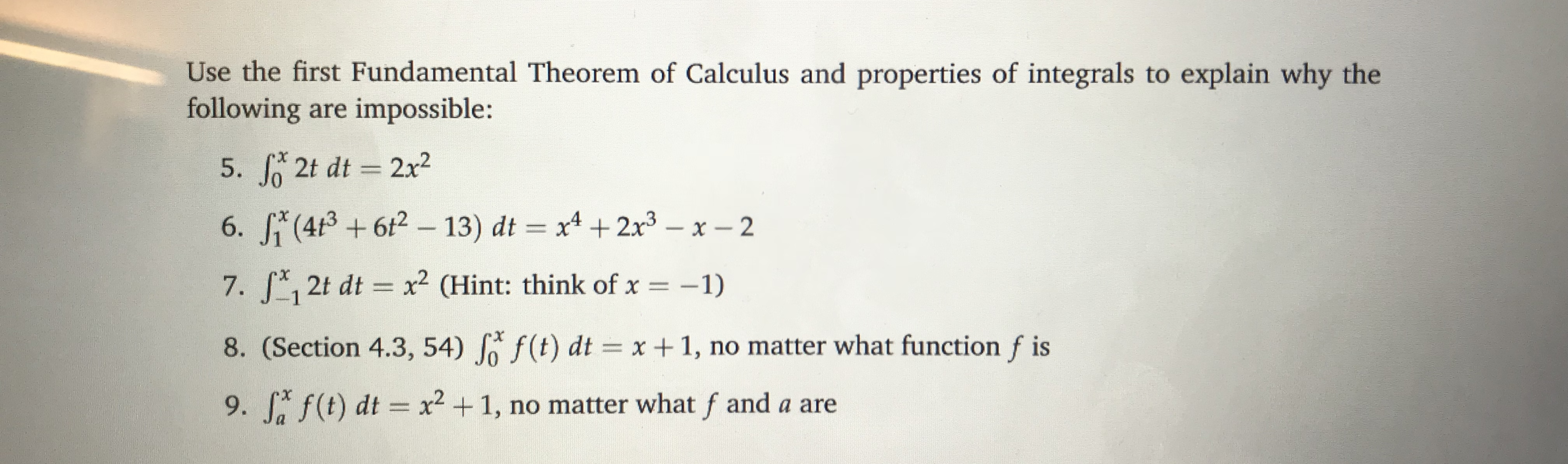# Use the first Fundamental Theorem of Calculus and properties of integrals to explain why the following are impossible: 5. J 2t dt 2x2 7. 12t dt 2 CHint: think of x-1) 8. (Section 4.3, 54) Jo f (t) dtx1, no matter what function f is 9, f,f(t) dt x2 + 1, no matter what f and a are

Questionhelp_outlineImage TranscriptioncloseUse the first Fundamental Theorem of Calculus and properties of integrals to explain why the following are impossible: 5. J 2t dt 2x2 7. 12t dt 2 CHint: think of x-1) 8. (Section 4.3, 54) Jo f (t) dtx1, no matter what function f is 9, f,f(t) dt x2 + 1, no matter what f and a are fullscreen